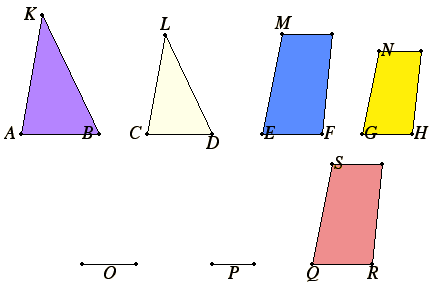# Proposition 22

If four straight lines are proportional, then the rectilinear figures similar and similarly described upon them are also proportional; and, if the rectilinear figures similar and similarly described upon them are proportional, then the straight lines are themselves also proportional.

Let the four straight lines AB, CD, EF, and GH be proportional, so that AB is to CD as EF is to GH. Let the similar and similarly situated rectilinear figures KAB and LCD be described on AB and CD, and the similar and similarly situated rectilinear figures MF and NH be described on EF and GH.

I say that KAB is to LCD as MF is to NH.

VI.11

Take a third proportional O to AB and CD, and a third proportional P to EF and GH.Then since AB is to CD as EF is to GH, therefore CD is to O as GH is to P. Therefore, ex aequali, AB is to O as EF is to P.

But AB is to O as KAB is to LCD, and EF is to P as MF is to NH, therefore KAB is to LCD also as MF is to NH.

Next, let KAB be to LCD as MF is to NH.

I say also that AB is to CD as EF is to GH.

For, if EF is not to GH as AB is to CD, let EF be to QR as AB is to CD. Describe the rectilinear figure SR similar and similarly situated to either of the two MF or NH on QR.

Above

Since then AB is to CD as EF is to QR, and there have been described on AB and CD the similar and similarly situated figures KAB and LCD, and on EF and QR the similar and similarly situated figures MF and SR, therefore KAB is to LCD as MF is to SR.

V.11

But also, by hypothesis, KAB is to LCD as MF is to NH, therefore also MF is to SR as MF is to NH.

V.9

Therefore MF has the same ratio to each of the figures NH and SR, therefore NH equals SR.

But it is also similar and similarly situated to it, therefore GH equals QR.

And, since AB is to CD as EF is to QR, while QR equals GH, therefore AB is to CD as EF is to GH.

Therefore, if four straight lines are proportional, then the rectilinear figures similar and similarly described upon them are also proportional; and, if the rectilinear figures similar and similarly described upon them are proportional, then the straight lines are themselves also proportional.

Q.E.D.

## Guide

There is a missing step near the end of the proof, namely, the justification of the statement that GH equals QR is missing. Just before it we have NH and SR are similar equal rectilinear figures, and we want to conclude the corresponding sides GH and QR are equal. The needed demonstration is not difficult to supply.

#### Use of this proposition

This proposition is used in the proofs of several propositions in Book X and in XII.4 in Book XII.